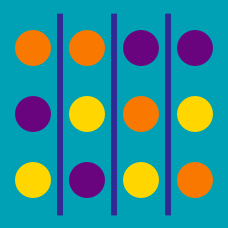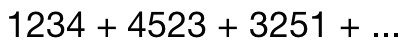Probability

# Permutations: Level 2 Challenges

If all words formed using only letters of the word "RANK" exactly once are arranged alphabetically. Find the position of the word RANK.

How many different positive integers exist between $10^{6}$ and $10^{7}$ the sum of whose digits is equal to 2?

How many 3 digit numbers are there, such that the hundreds digit is strictly smaller than the tens digit, which is strictly smaller than the units digit?

Can you solve this without listing?

Continued from ...Find the sum of all 4-digit numbers formed by using the digits 1, 2, 3, 4, 5 and where no digit is to be repeated in any 4-digit number.

What is the number of integers greater than 6000 that can be formed using the digits 3,5,6,7 and 8 without repetition?

×